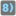• # Possible error in Check

## Question related to missionFriendly Number

I am still not complete with my solution, but when I run the Check, I get a fail on 2.Edges Test 5.5

friendly_number(4294967297, base=2, powers=["p0","p1","p2","p3","p4","p5","p6","p7","p8","p9","p10","p11","p12","p13","p14","p15","p16","p17","p18","p19","p20","p21","p22","p23","p24","p25","p26","p27","p28","p29","p30","p31"])

where the base is 2. My answer is "10p31" but the preferred answer is "2p31". It is my opinion that an answer for a number converted to binary (base 2) can never have digits other than "0" and "1" so I assert my answer (10(binary)=2(decimal)) is actually the correct one. I don't want to add more special case text to my code since I believe it handles this test correctly. Does the mission expect the final answer to be converted back to decimal? That does not seem to be the expectation on the other asserts. For example:

assert friendly_number(1024000000, base=1024, suffix='iB') == '976MiB' (976 is the decimal value of a single digit in base 1024)

I have attached the text of my code if you cannot access the code in my account (I don't know any other way to pass my code over).14